# ML 107: Evasion Attack with SecML (40 pts extra)

## What You Need

• A Web browser

## Purpose

To practice using SecML, an open-source Python library for the security evaluation of Machine Learning algorithms.

In a browser, go to

From the menu, click File, "New notebook".

## Installing SecML

Execute these commands:
```!pip install secml import secml ```
The library installs, as shown below.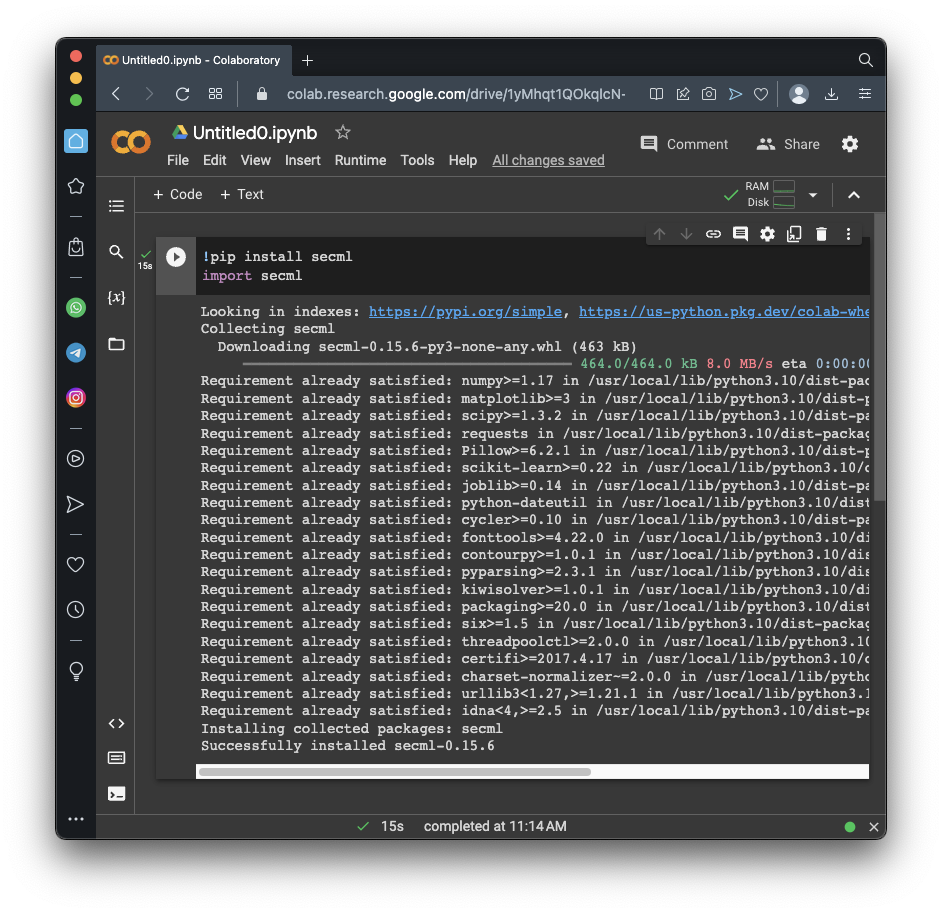## Preparing a Dataset

Execute these commands to create a simple, artificial dataset consisting of three groups of points in a plane.
```random_state = 999 n_features = 2 # Number of features n_samples = 1250 # Number of samples centers = [[-2, 0], [2, -2], [2, 2]] # Centers of the clusters cluster_std = 0.8 # Standard deviation of the clusters from secml.data.loader import CDLRandomBlobs dataset = CDLRandomBlobs(n_features=n_features, centers=centers, cluster_std=cluster_std, n_samples=n_samples, random_state=random_state).load() n_tr = 1000 # Number of training set samples n_ts = 250 # Number of test set samples # Split in training and test from secml.data.splitter import CTrainTestSplit splitter = CTrainTestSplit( train_size=n_tr, test_size=n_ts, random_state=random_state) tr, ts = splitter.split(dataset) # Normalize the data from secml.ml.features import CNormalizerMinMax nmz = CNormalizerMinMax() tr.X = nmz.fit_transform(tr.X) ts.X = nmz.transform(ts.X) from secml.figure import CFigure # Only required for visualization in notebooks %matplotlib inline fig = CFigure(width=5, height=5) # Convenience function for plotting a dataset fig.sp.plot_ds(tr) fig.show() ```
As shown below, you see three categories of dots, shown in different colors.

The task of this model is to sort the dots into their categories.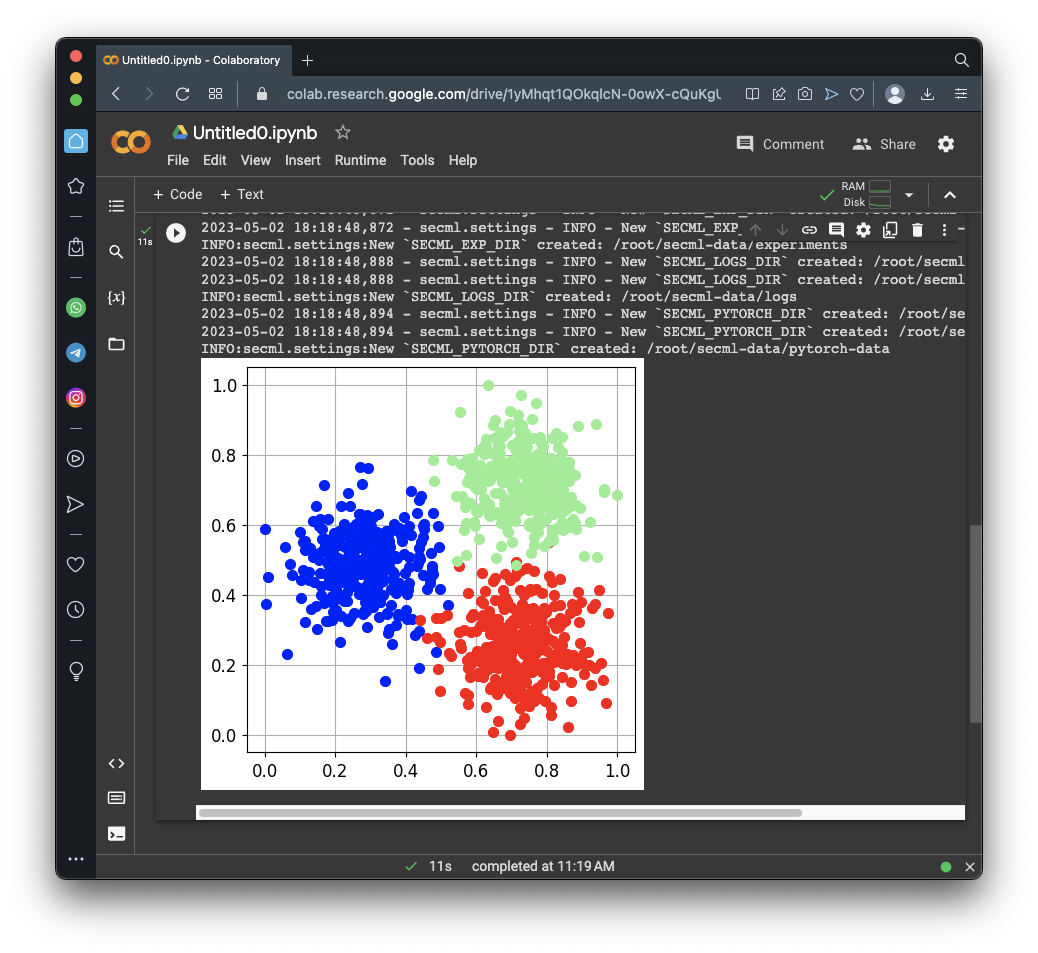## Support Vector Machines (SVMs)

Consider the classification shown below, from An Idiot’s guide to Support vector machines (SVMs).

The task is to saparate the red dots from the green dots. Either of the lines shown below correctly classify all the dots.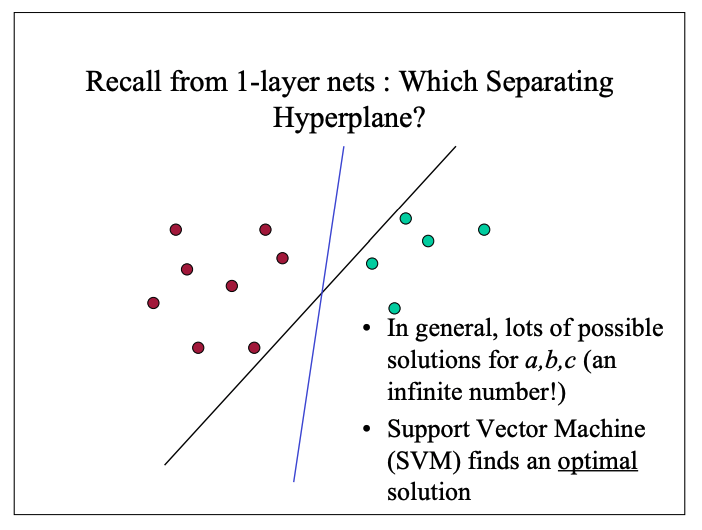A SVM chooses the line that maximizes the "margin" or "street width" between the categories, as shown below.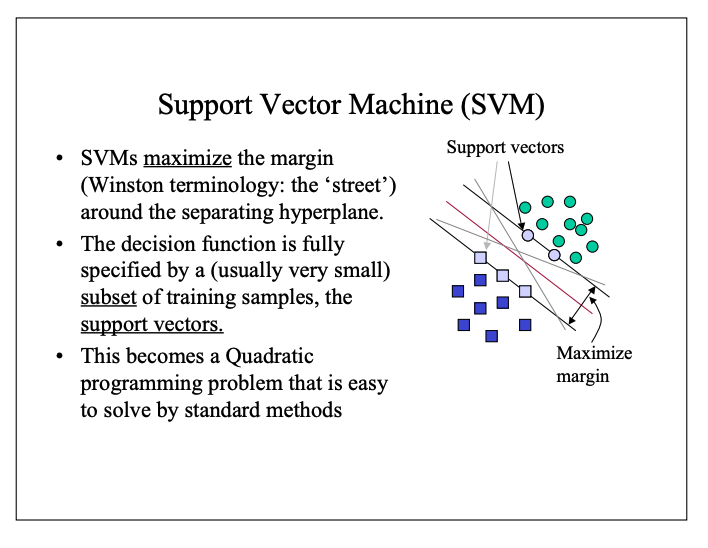## Creating and Training the Model

Execute these commands to create and train the SVM model:
```# Creation of the multiclass classifier from secml.ml.classifiers import CClassifierSVM from secml.ml.kernels import CKernelRBF svm = CClassifierSVM(kernel=CKernelRBF()) # Parameters for the Cross-Validation procedure xval_params = {'C': [0.1, 1, 10], 'kernel.gamma': [1, 10, 100]} # Let's create a 3-Fold data splitter from secml.data.splitter import CDataSplitterKFold xval_splitter = CDataSplitterKFold(num_folds=3, random_state=random_state) # Metric to use for training and performance evaluation from secml.ml.peval.metrics import CMetricAccuracy metric = CMetricAccuracy() # Select and set the best training parameters for the classifier print("Estimating the best training parameters...") best_params = svm.estimate_parameters( dataset=tr, parameters=xval_params, splitter=xval_splitter, metric=metric, perf_evaluator='xval' ) print("The best training parameters are: ", [(k, best_params[k]) for k in sorted(best_params)]) # We can now fit the classifier svm.fit(tr.X, tr.Y) # Compute predictions on a test set y_pred = svm.predict(ts.X) # Evaluate the accuracy of the classifier acc = metric.performance_score(y_true=ts.Y, y_pred=y_pred) print("Accuracy on test set: {:.2%}".format(acc)) ```
As shown below, the model has a 98.8% accuracy.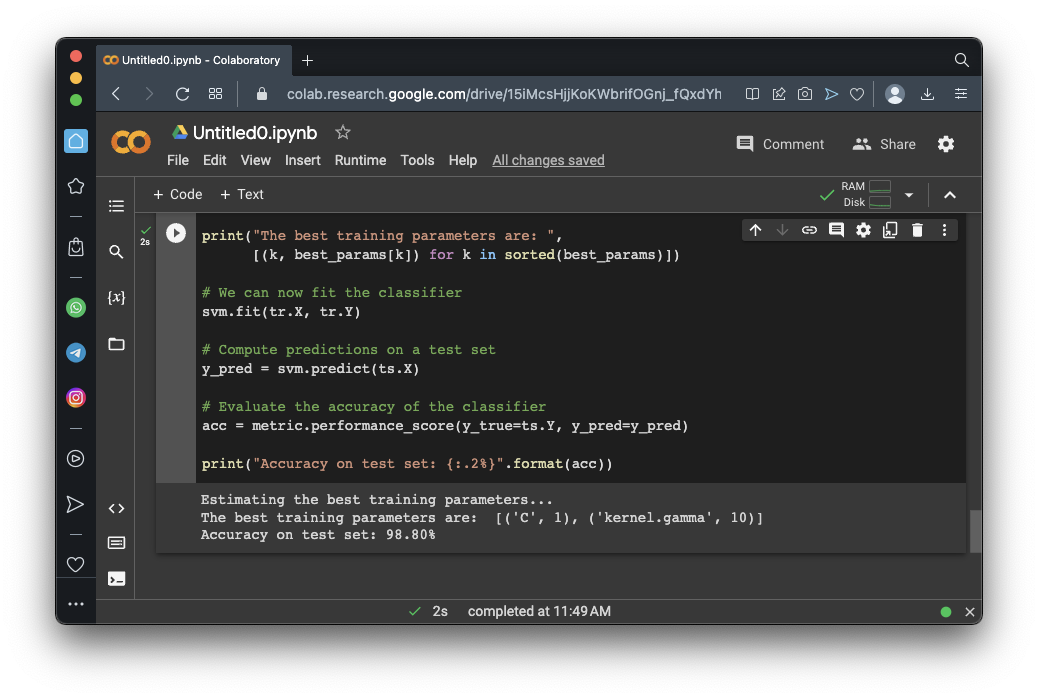## Visualizing the Results

Execute these commands to see a pretty cart showing the regions the model classifies data into:
```fig = CFigure(width=5, height=5) # Convenience function for plotting the decision function of a classifier fig.sp.plot_decision_regions(svm, n_grid_points=200) fig.sp.plot_ds(ts) fig.sp.grid(grid_on=False) fig.sp.title("Classification regions") fig.sp.text(0.01, 0.01, "Accuracy on test set: {:.2%}".format(acc), bbox=dict(facecolor='white')) fig.show() ```
As shown below, the model draws curves between the regions, more or less the same way a human would.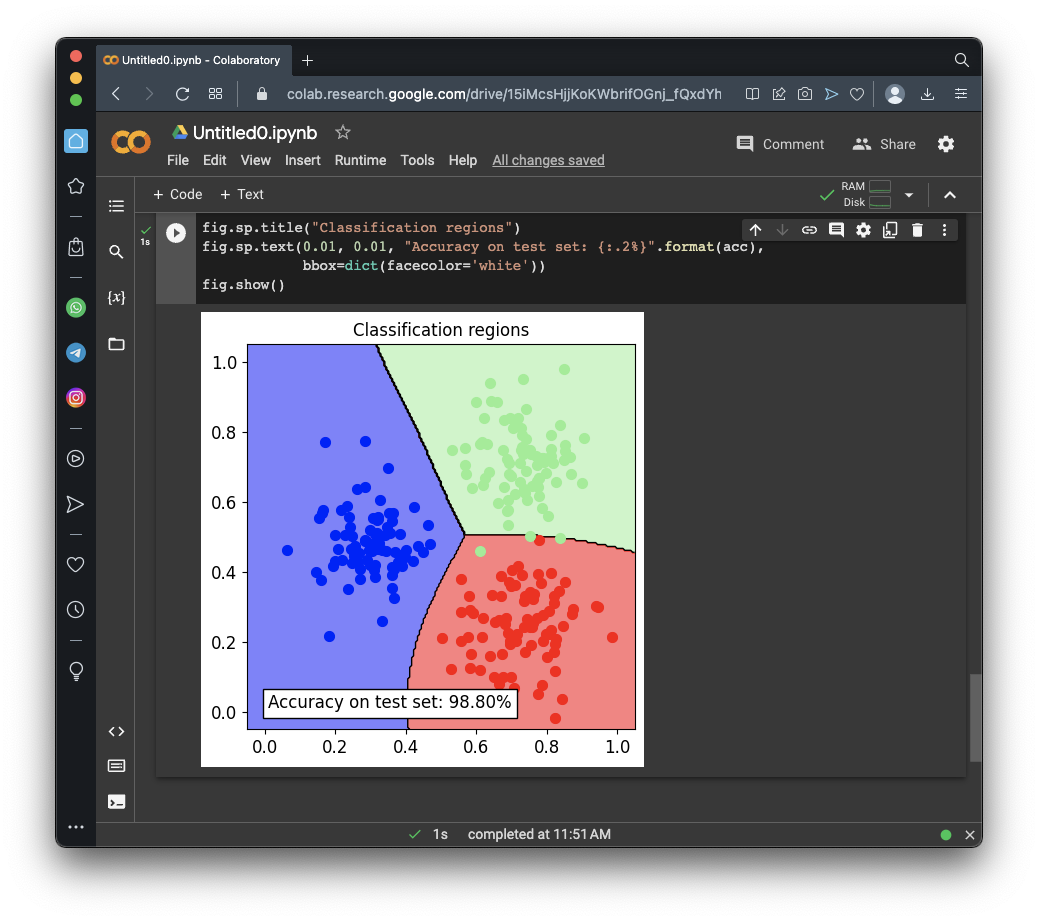## Understanding Evasion Attacks

An evasion attack sends a model crafted inputs which fool it, even though it might look fine to a human observer.

For example, adding noise to a panda image makes a machine learning model misidentify it as a gibbon, as shown below.## Performing an Evasion Attack

In this attack, we'll start from the test set element 5, which is at coordinates (0.76, 0.34) in the red region. We'll move that point, using a "gradient-based maximum-confidence" algorithm to quickly move it to a value that causes the model to misidentify it.

Execute these commands:

```x0, y0 = ts[5, :].X, ts[5, :].Y # Initial sample--X is location, Y is classification noise_type = 'l2' # Type of perturbation 'l1' or 'l2' dmax = 0.4 # Maximum perturbation lb, ub = 0, 1 # Bounds of the attack space. Can be set to `None` for unbounded y_target = None # Move until the model gets any wrong answer # Should be chosen depending on the optimization problem solver_params = { 'eta': 0.3, 'eta_min': 0.1, 'eta_max': None, 'max_iter': 100, 'eps': 1e-4 } from secml.adv.attacks.evasion import CAttackEvasionPGDLS pgd_ls_attack = CAttackEvasionPGDLS( classifier=svm, double_init_ds=tr, double_init=False, distance=noise_type, dmax=dmax, lb=lb, ub=ub, solver_params=solver_params, y_target=y_target) # Run the evasion attack on x0 y_pred_pgdls, _, adv_ds_pgdls, _ = pgd_ls_attack.run(x0, y0) print("Original x:", x0, "label: ", y0.item()) print("Final x: ", adv_ds_pgdls.X, "label: ", y_pred_pgdls.item(), ) print("Number of classifier gradient evaluations: {:}" "".format(pgd_ls_attack.grad_eval)) fig = CFigure(width=5, height=5) fig.sp.plot_decision_regions(svm, n_grid_points=200) fig.sp.plot_ds(ts) fig.sp.grid(grid_on=False) fig.sp.plot_path(x0.append(adv_ds_pgdls.X, axis=0)) fig.show() ```
As shown below, the evasion attack moved point up from the red region into the green region.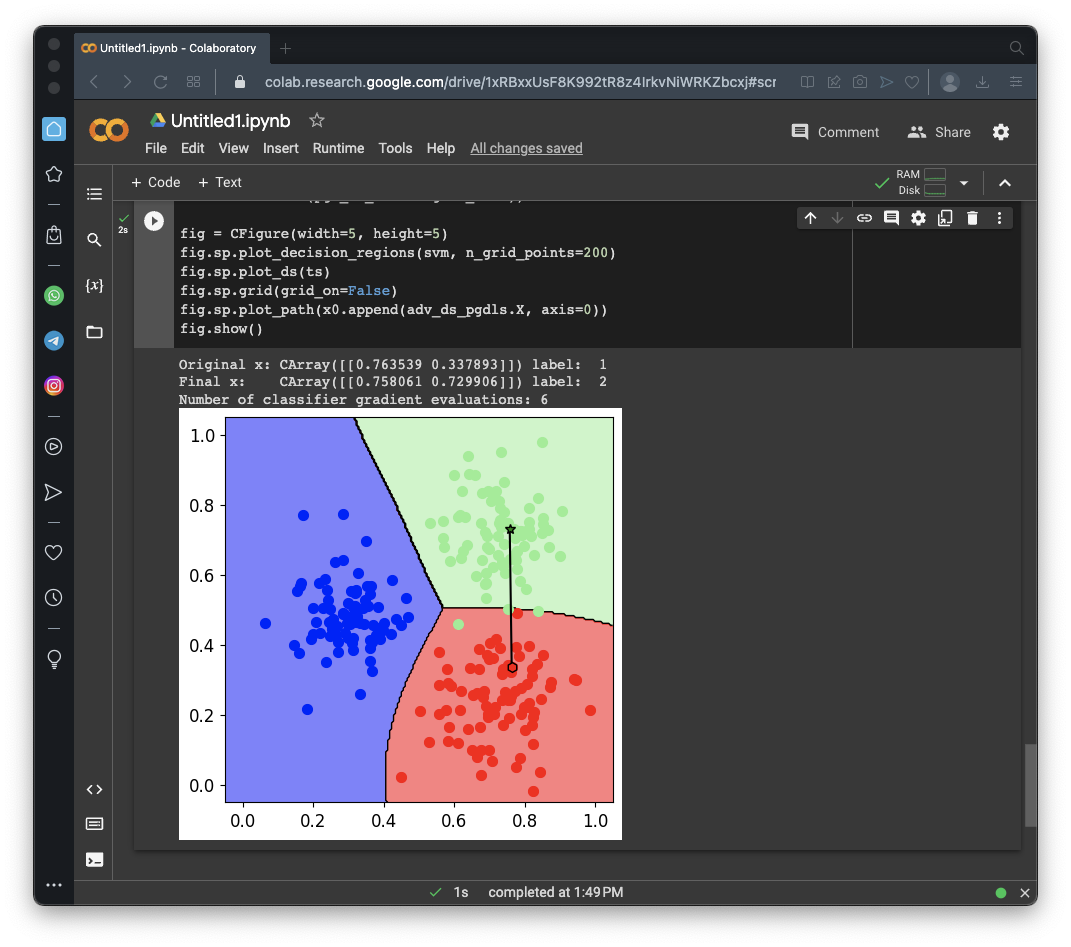## Security Evaluation

Now we'll run a test to see how much the performance of the model degrades under various attacks. We'll vary the maximum perturbation (how far we can move a dot) from 0 to 1.

Execute these commands. This will take several minutes to run.

```# Perturbation levels to test from secml.array import CArray e_vals = CArray.arange(start=0, step=0.1, stop=1.1) from secml.adv.seceval import CSecEval sec_eval = CSecEval( attack=pgd_ls_attack, param_name='dmax', param_values=e_vals) # Run the security evaluation using the test set print("Running security evaluation...") sec_eval.run_sec_eval(ts) from secml.figure import CFigure fig = CFigure(height=5, width=5) # Convenience function for plotting the Security Evaluation Curve fig.sp.plot_sec_eval( sec_eval.sec_eval_data, marker='o', label='SVM RBF', show_average=True) ```
As shown below, the accuracy falls to 50% at a dmax of about 0.23.## Flag ML 107.1: Title (10 pts)

The flag is covered by a green rectangle in the image above.

## Flag ML 107.2: Evasion from Point 0 (5 pts)

Perform the same evasion attack starting from element zero in the training set, not element 5.

The flag is covered by a green rectangle in the image below.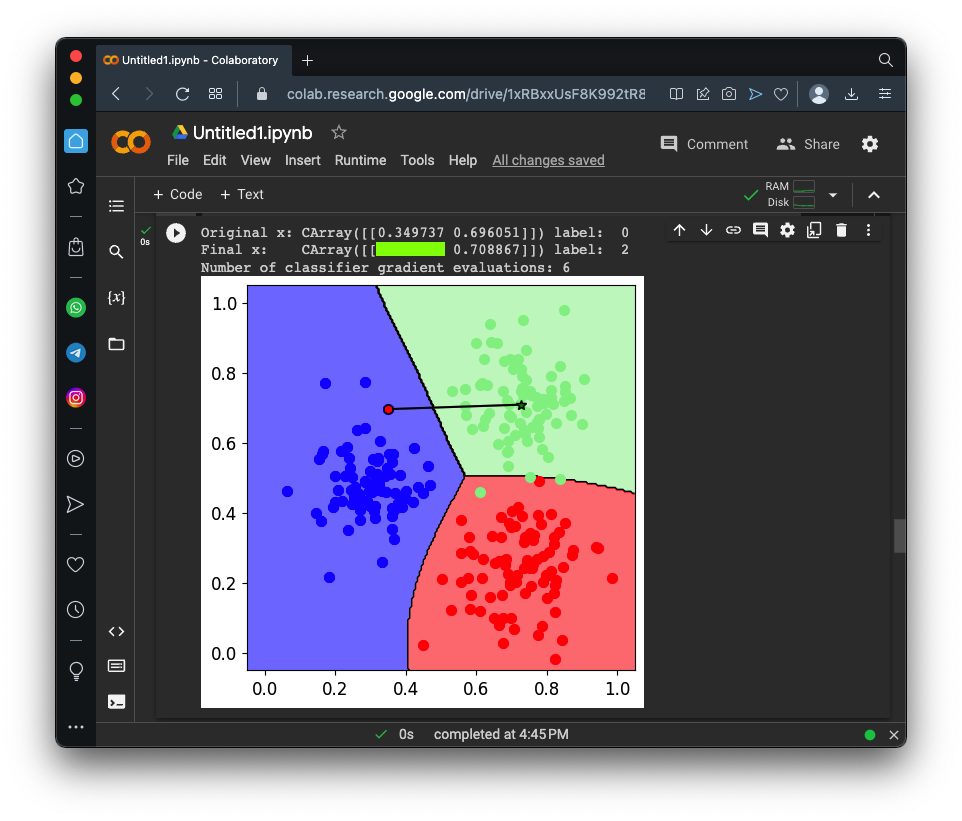## Flag ML 107.3: Green High (10 pts)

Move the green cluster higher by 0.5.

Train the model on that data, and perform the same evasion attack starting from element 1 in the training set.

The flag is covered by a green rectangle in the image below.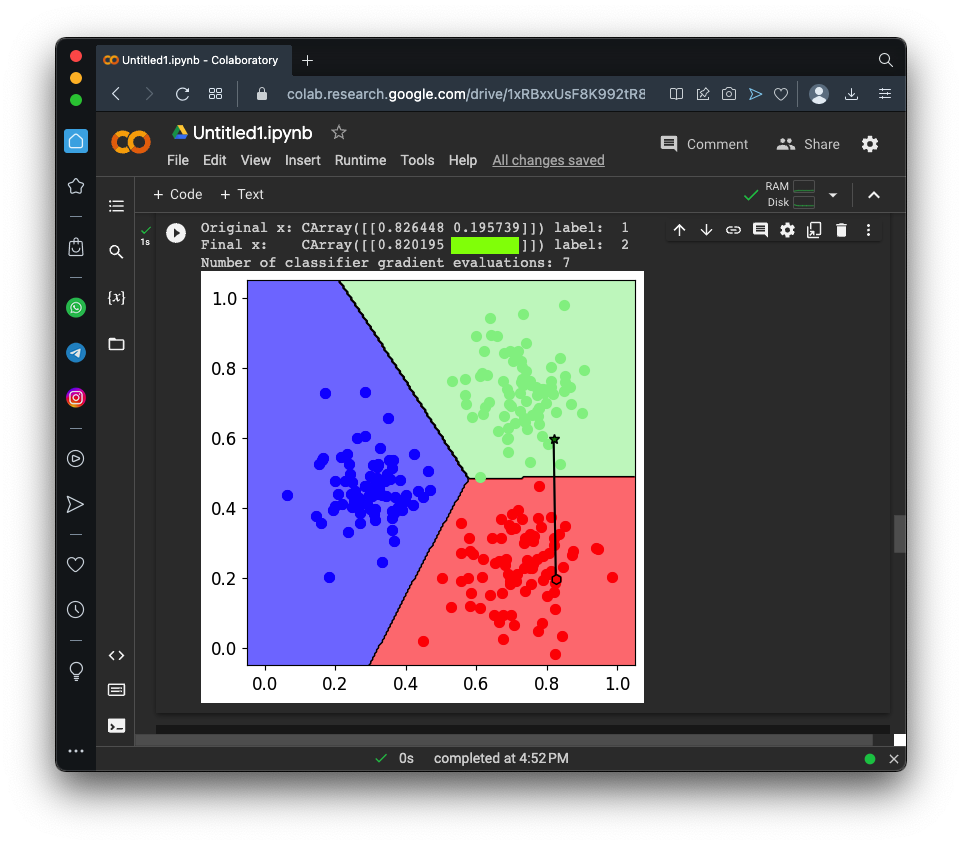## Flag ML 107.4: Noisier Problem (15 pts)

Start from the original model and data. Make these changes:
• Move the red cluster to the left by 0.2.
• Change the standard deviation to 1.1
• Change the number of training set samples to 2000
• Change the number of test samples to 500
• Start the evasion attack at element 10 in the training set
The evasion attack results are as shown below.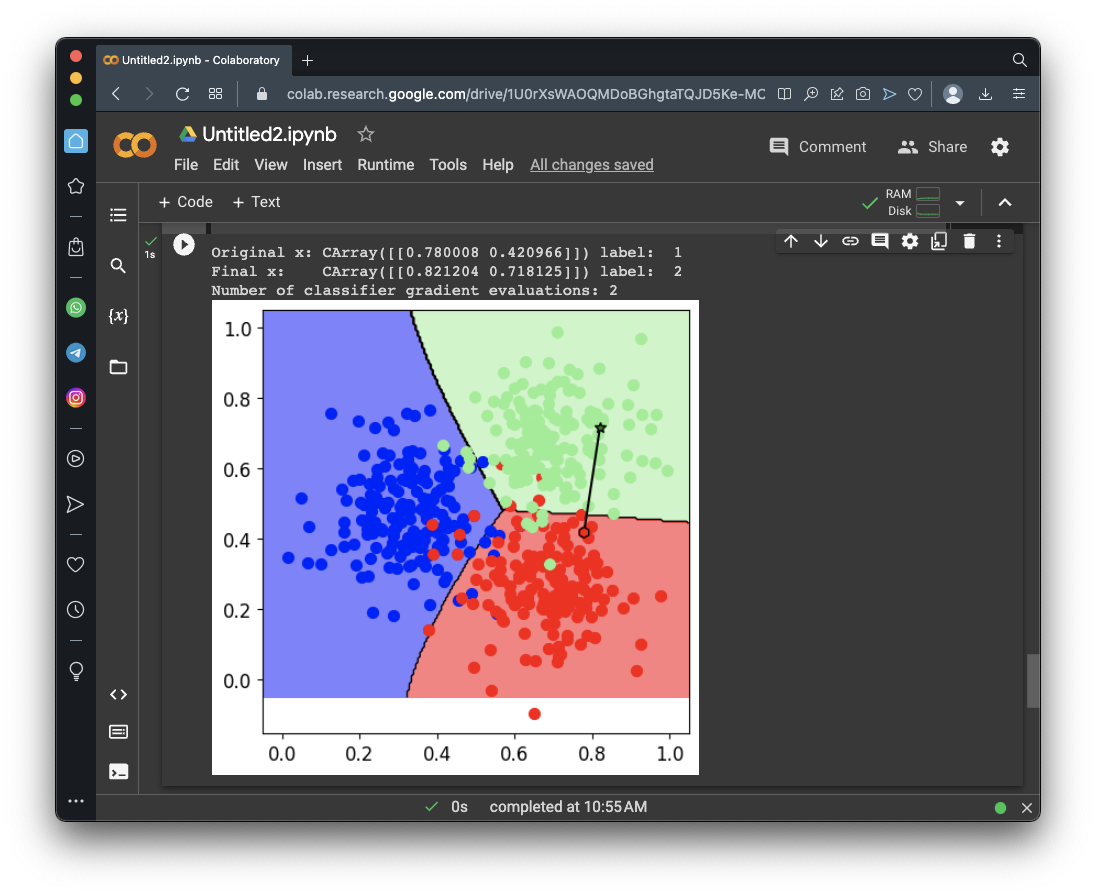Perform the security evaluation, as you did above.

The flag is covered by a green rectangle in the image below.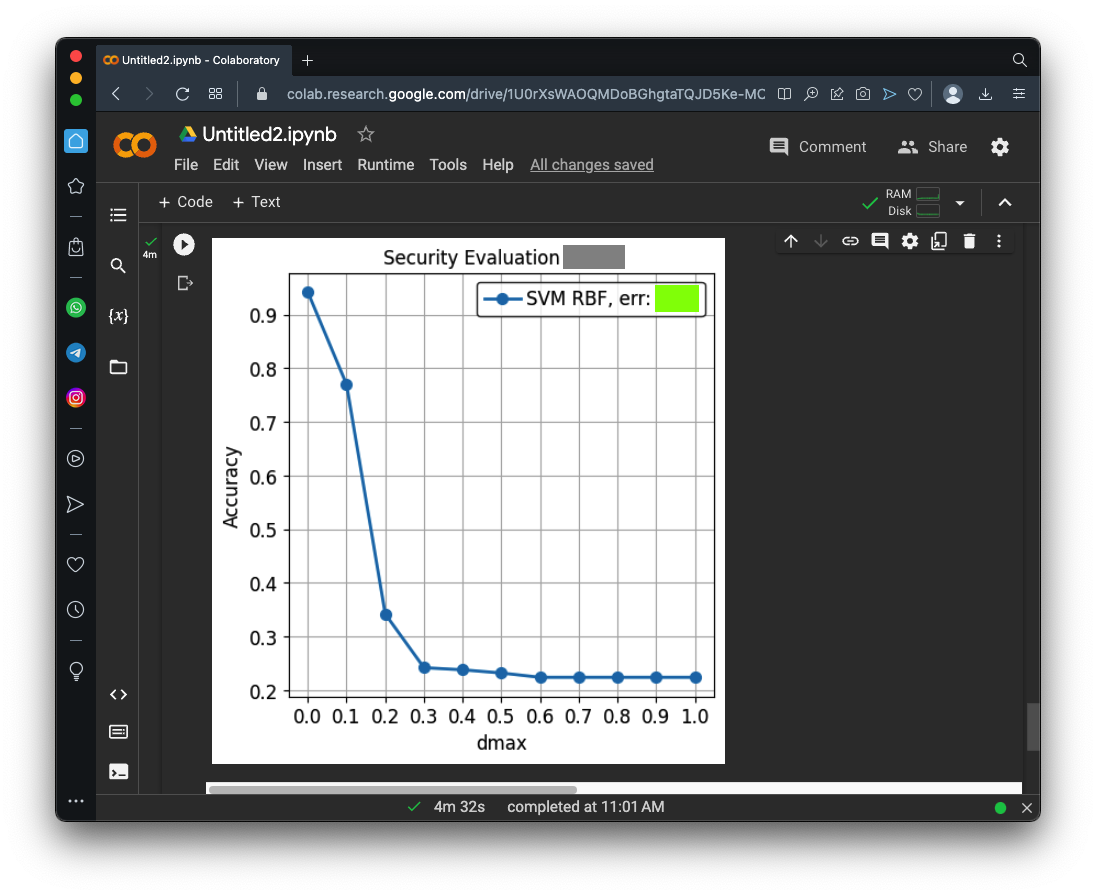## Sources

SecML: Secure and Explainable Machine Learning in Python
Training of Classifiers and Visualization of Results
An Idiot's guide to Support vector machines (SVMs)
Evasion attacks on Machine Learning (or 'Adversarial Examples')

Posted 5-2-23